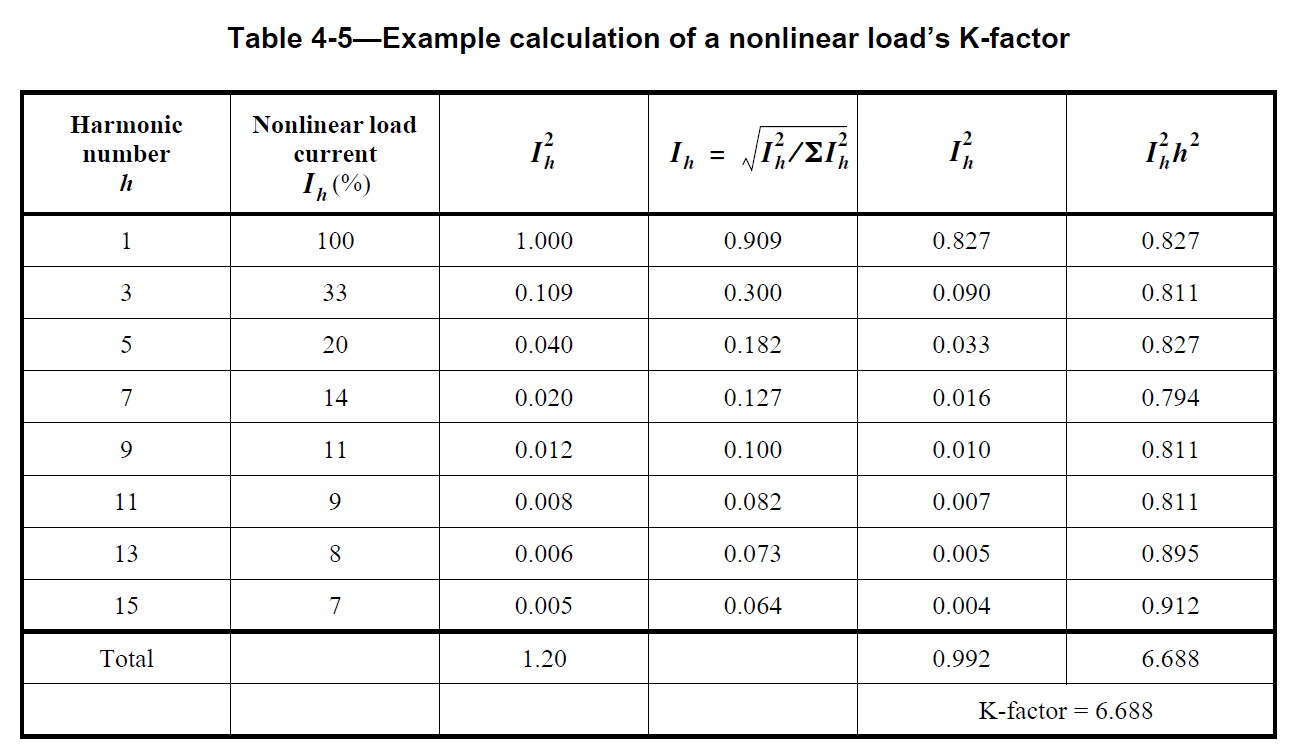### What is Transformer K-Factor Rating

A conventional transformer has restricted use in an environment where non-linear loads are utilized, unbalanced input voltage or load current exceeds 5% total harmonic distortion.

K-factor rating is a value used to determine how much harmonic current a transformer can handle without exceeding its maximum temperature rise level. K-factor values range from 1 to 50. K-factor of 1 is used for linear loads only, and a K-factor of 50 is used for the harshest harmonic environment possible.

The K-factor is a number derived from a numerical calculation based on the summation of harmonic currents generated by the non-linear load. The higher the K-factor, the more significant the harmonic current content. Details of the calculation method can be found in IEEE Standard 1100-2005.

### Derating of Transformer With No K-Factor Rating

At present, most transformers in service do not have an assigned K-factor rating than transformers that do. Even with newly installed transformers, Electrical Engineers miss incorporating the effects of harmonics when sizing their transformers. Despite these transformers having been providing service adequately, this does not negate the fact that harmonic distortion causes increased heating in transformers.

Technology is changing. More non-linear loads such as variable speed drives, computers, electronic ballasts, electronics equipment, etc are being connected to existing facilities giving consideration to the fundamental frequency loads only but not harmonics when performing equipment adequacy verification.

Transformers that are not K-factor rated to be used with nonlinear loads are generally restricted to use on circuits with the following characteristics, which are specified by IEEE Std C57-12.00™ (for liquid-immersed) and IEEE Std C57.12.01™ (for dry-type) transformers:

1. Approximately sinusoidal, balanced input voltage, and
2. Full-load current that does not exceed 5.0% of total harmonic distortion

These limitations are primarily due to eddy currents induced in both the windings and structural components that increase losses and can cause overheating. Transformer derating is necessary since typical power and general-purpose are not designed to supply non-linear loads.

### Calculation of Transformer With No K-Factor Rating

Transformers that are not K-factor rated have lower investment costs than K-rated transformers. How is the K-factor rating of a transformer calculated to ensure that the design is still economical? IEEE Standard 1100-2005 provides the methodology for determining the K-factor rating of a transformer when the load's harmonics are known.Categories: